# Window function

A function used to restrict consideration of an arbitrary function or signal in some way. The terms time-frequency localization, time localization or frequency localization are often used in this context. For instance, the windowed Fourier transform is given by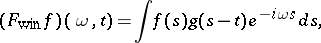whereis a suitable window function. Quite often, scaled and translated versions of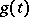are considered at the same time, [a1], [a3]. An example is the Gabor transform. (See also Balian–Low theorem; Calderón-type reproducing formula.) Such window functions are also used in numerical analysis.

More specifically, the phrase window function refers to the functionthat equalson the interval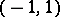and zero elsewhere (at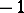and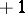it is arbitrarily defined, usually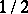or). This function, as well as its scaled and translated versions, is also called the rectangle function or pulse function [a2], pp. 30, 35, 60, 61. However, the phrase "pulse function" is also sometimes used for the delta-function, see also Transfer function.

The Fourier transform of the specific rectangle function(with) is the functiona version of the sinc function (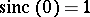,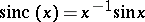for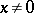), see [a2], pp. 61, 104. In terms of the Heaviside function(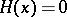for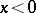,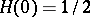,for),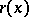is given by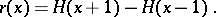There is also a relation with the Dirac delta-function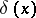: